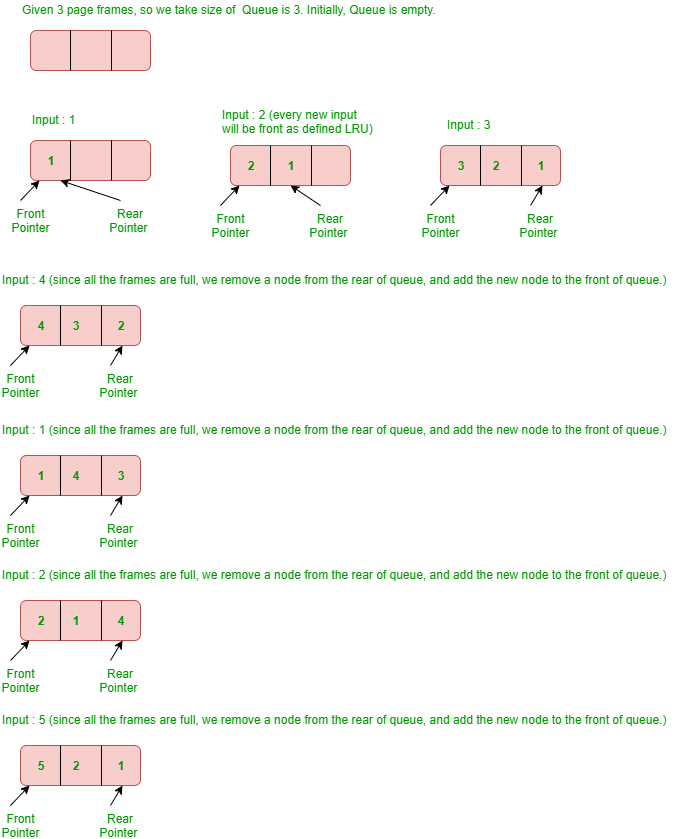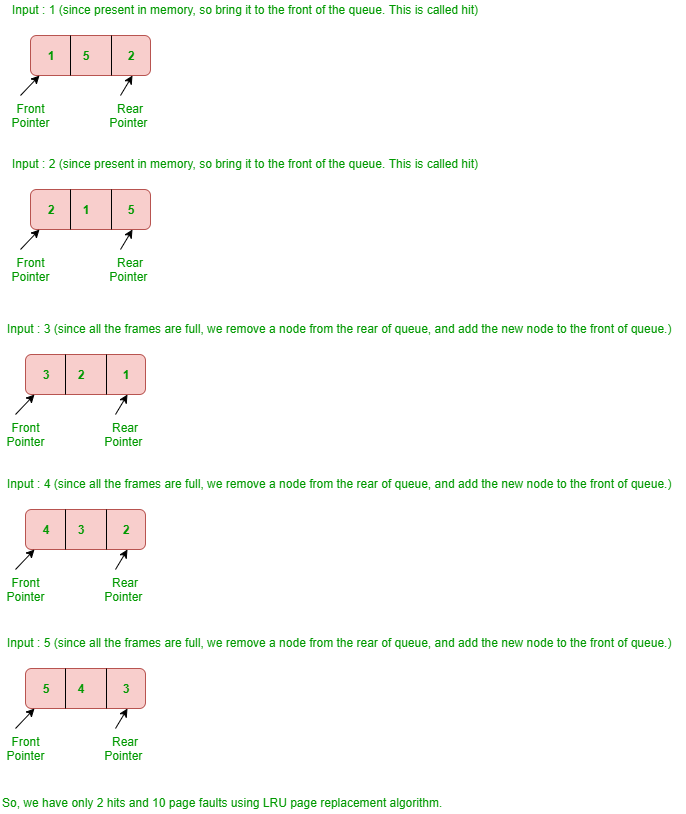# Python – LRU Cache

LRU Cache is the least recently used cache which is basically used for Memory Organization. In this, the elements come as First in First Out format. We are given total possible page numbers that can be referred to. We are also given cache (or memory) size (Number of page frames that cache can hold at a time). The LRU caching scheme is to remove the least recently used frame when the cache is full and a new page is referenced which is not there in the cache. There are generally two terms use with LRU Cache, let’s see them –

• Page hit: If the required page is found in the main memory then it is a page hit.
• Page Fault: If the required page is not found in the main memory then page fault occurs.

When a page is referenced, the required page may be in the memory. If it is in the memory, we need to detach the node of the list and bring it to the front of the queue.
If the required page is not in memory, we bring that in memory. In simple words, we add a new node to the front of the queue and update the corresponding node address in the hash. If the queue is full, i.e. all the frames are full, we remove a node from the rear of the queue, and add the new node to the front of the queue.

Example – Consider the following reference string :

`1, 2, 3, 4, 1, 2, 5, 1, 2, 3, 4, 5`

Find the number of page faults using least recently used (LRU) page replacement algorithm with 3 page frames.
Explanation –LRU Cache Using Python
You can implement this with the help of the queue. In this, we have used Queue using the linked list. Run the given code in Pycharm IDE.

 `import` `time ` `  `  `     `  `class` `Node: ` `     `  `    ``# Nodes are represented in n ` `    ``def` `__init__(``self``, key, val): ` `        ``self``.key ``=` `key ` `        ``self``.val ``=` `val ` `        ``self``.``next` `=` `None` `        ``self``.prev ``=` `None` `  `  `  `  `class` `LRUCache: ` `    ``cache_limit ``=` `None` `     `  `    ``# if the DEBUG is TRUE then it ` `    ``# will execute ` `    ``DEBUG ``=` `False` `  `  `    ``def` `__init__(``self``, func): ` `        ``self``.func ``=` `func ` `        ``self``.cache ``=` `{} ` `        ``self``.head ``=` `Node(``0``, ``0``) ` `        ``self``.tail ``=` `Node(``0``, ``0``) ` `        ``self``.head.``next` `=` `self``.tail ` `        ``self``.tail.prev ``=` `self``.head ` `  `  `    ``def` `__call__(``self``, ``*``args, ``*``*``kwargs): ` `         `  `        ``# The cache presents with the help ` `        ``# of Linked List ` `        ``if` `args ``in` `self``.cache: ` `            ``self``.llist(args) ` `             `  `            ``if` `self``.DEBUG ``=``=` `True``: ` `                ``return` `f``'Cached...{args}\n{self.cache[args]}\nCache: {self.cache}'` `            ``return` `self``.cache[args] ` `  `  `        ``# The given cache keeps on moving. ` `        ``if` `self``.cache_limit ``is` `not` `None``: ` `             `  `            ``if` `len``(``self``.cache) > ``self``.cache_limit: ` `                ``n ``=` `self``.head.``next` `                ``self``._remove(n) ` `                ``del` `self``.cache[n.key] ` `  `  `        ``# Compute and cache and node to see whether  ` `        ``# the following element is present or not  ` `        ``# based on the given input. ` `        ``result ``=` `self``.func(``*``args, ``*``*``kwargs) ` `        ``self``.cache[args] ``=` `result ` `        ``node ``=` `Node(args, result) ` `        ``self``._add(node) ` `         `  `        ``if` `self``.DEBUG ``=``=` `True``: ` `            ``return` `f``'{result}\nCache: {self.cache}'` `        ``return` `result ` `  `  `    ``# Remove from double linked-list - Node. ` `    ``def` `_remove(``self``, node): ` `        ``p ``=` `node.prev ` `        ``n ``=` `node.``next` `        ``p.``next` `=` `n ` `        ``n.prev ``=` `p ` `  `  `    ``# Add to double linked-list - Node. ` `    ``def` `_add(``self``, node): ` `        ``p ``=` `self``.tail.prev ` `        ``p.``next` `=` `node ` `        ``self``.tail.prev ``=` `node ` `        ``node.prev ``=` `p ` `        ``node.``next` `=` `self``.tail ` `  `  `    ``# Over here the result task is being done  ` `    ``def` `llist(``self``, args): ` `        ``current ``=` `self``.head ` `         `  `        ``while` `True``: ` `             `  `            ``if` `current.key ``=``=` `args: ` `                ``node ``=` `current ` `                ``self``._remove(node) ` `                ``self``._add(node) ` `                 `  `                ``if` `self``.DEBUG ``=``=` `True``: ` `                    ``del` `self``.cache[node.key]   ` `                    ``self``.cache[node.key] ``=` `node.val  ` `                ``break` `             `  `            ``else``: ` `                ``current ``=` `current.``next` `  `  `  `  `# Default Debugging is FALSE. For  ` `# execution of DEBUG is set to TRUE ` `LRUCache.DEBUG ``=` `True` `  `  `# The DEFAULT test limit is NONE. ` `LRUCache.cache_limit ``=` `3` `  `  ` `  `@LRUCache` `def` `ex_func_01(n): ` `    ``print``(f``'Computing...{n}'``) ` `    ``time.sleep(``1``) ` `    ``return` `n ` `  `  `  `  `print``(f``'\nFunction: ex_func_01'``) ` `print``(ex_func_01(``1``)) ` `print``(ex_func_01(``2``)) ` `print``(ex_func_01(``3``)) ` `print``(ex_func_01(``4``)) ` `print``(ex_func_01(``1``)) ` `print``(ex_func_01(``2``)) ` `print``(ex_func_01(``5``)) ` `print``(ex_func_01(``1``)) ` `print``(ex_func_01(``2``)) ` `print``(ex_func_01(``3``)) ` `print``(ex_func_01(``4``)) ` `print``(ex_func_01(``5``)) `

Output:

```Function: ex_func_01
Computing...1
1
Cache: {(1,): 1}
Computing...2
2
Cache: {(1,): 1, (2,): 2}
Computing...3
3
Cache: {(1,): 1, (2,): 2, (3,): 3}
Computing...4
4
Cache: {(1,): 1, (2,): 2, (3,): 3, (4,): 4}
Cached...(1,)
1
Cache: {(2,): 2, (3,): 3, (4,): 4, (1,): 1}
Cached...(2,)
2
Cache: {(3,): 3, (4,): 4, (1,): 1, (2,): 2}
Computing...5
5
Cache: {(4,): 4, (1,): 1, (2,): 2, (5,): 5}
Cached...(1,)
1
Cache: {(4,): 4, (2,): 2, (5,): 5, (1,): 1}
Cached...(2,)
2
Cache: {(4,): 4, (5,): 5, (1,): 1, (2,): 2}
Computing...3
3
Cache: {(5,): 5, (1,): 1, (2,): 2, (3,): 3}
Computing...4
4
Cache: {(1,): 1, (2,): 2, (3,): 3, (4,): 4}
Computing...5
5
Cache: {(2,): 2, (3,): 3, (4,): 4, (5,): 5}```

My Personal Notes arrow_drop_upCheck out this Author's contributed articles.

If you like GeeksforGeeks and would like to contribute, you can also write an article using contribute.geeksforgeeks.org or mail your article to contribute@geeksforgeeks.org. See your article appearing on the GeeksforGeeks main page and help other Geeks.

Please Improve this article if you find anything incorrect by clicking on the "Improve Article" button below.

Article Tags :

1

Please write to us at contribute@geeksforgeeks.org to report any issue with the above content.